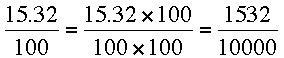SEARCH HOMEMath Central Quandaries & QueriesQuestion from Abby, a student: write 43.75% as a fraction or mixed number in lowest terms.Hi Abby,

Percent means per one hundred. The prefix suffix cent comes from the Latin word centum which means one hundred. That's why we use the word cent for one one-hundredth of a dollar. Hence 15.32% is another way of writing 15.32/100. We usually don't write numbers that way, mixing decimal notation and common fraction notation in the same expression so multiply the numerator and denominator by 100 to getThe numerator and denominator are each divisible by 4 and thus, dividing each by 4 gives 338/2500. This fraction doesn't simplify any further and hence 15.32% expressed as a fraction in lowest terms is 338/2500.

Now try 43.75%,
PennyMath Central is supported by the University of Regina and The Pacific Institute for the Mathematical Sciences.